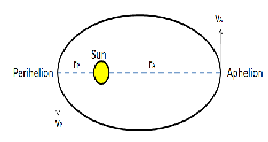# Out of aphelion and perihelion, where is the speed of the Earth greater and why?

## Question:

Out of aphelion and perihelion, where is the speed of the Earth greater and why?

## Kepler's Law

Kepler found important regularities in the motion of the planets around the sun, these regularities were summed under Kepler's Three Laws of Planetary Motion.

The terms perihelion and aphelion are different points on the earth's orbit around the sun.

• Perihelion is the point on the earth's orbit that is nearest to the sun.
• Aphelion is the point on the earth's orbit that is farthest from the sun.Let us see where the speed of the earth is greater.

The angular momentum of the earth about the sun is constant, so

{eq}mr_{A}v_{A} = mr_{P}v_{P} {/eq}

where m is the mass of the planet,

{eq}\Rightarrow r_{A}v_{A} = r_{P}v_{P} {/eq} is the areal velocity.{eq}\Rightarrow \frac{r_{A}}{r_{P}} = \frac{v_{P}}{v_{A}} {/eq}

Since {eq}r_{A}> r_{P} {/eq} as shown in Fig. 1

{eq}\therefore v_{P} > v_{A} {/eq}

{eq}\Rightarrow The{/eq} velocity of the earth is greater at the perihelion.